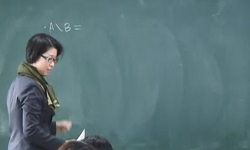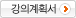### 주메뉴

### 해석학1

• 건국대학교
• 김영주• 주제분류
자연과학 >수학ㆍ물리ㆍ천문ㆍ지리 >수학
• 강의학기
2016년 1학기
• 조회수
7,444
• 평점
0/5.0 (2)
강의계획서Real and complex number system, basic topology, sequence, series, continuity, differentiation, and Riemann-Stieltjes integral.
The real number system#### 차시별 강의1.The real number system We introduce the concept of least upper bound property and identify the least upper bound for various subsets of the set real numbers.2.The real number system mathematical induction3.Equivalents of the least upper bound property We study important properties of the real number system deduced from the least upper bound property such as Archimedian property.4.Equivalents of the least upper bound property Least upper bound property of Real number5.Countable and uncountable sets We introduce the concept of countability and uncountability of various sets.6.Sequences of real numbers We study the metric structure of the real number system and define convergence of sequences. Further, we compute the limits of various sequences.7.Convergent sequences We study the metric structure of the real number system and define convergence of sequences. Further, we compute the limits of various sequences.8.Convergent sequences We study the metric structure of the real number system and define convergence of sequences. Further, we compute the limits of various sequences.9.Monotone sequences We prove the monotone convergence theorem and derive the nested interval property from it.10.Sequences and Bolzano-Weierstrass theorem We introduce the concept of limit points and prove Bolzano-Weierstrass theorem.11.Limit points We introduce the concept of limit points and prove Bolzano-Weierstrass theorem.12.Limit points We introduce the concept of limit points and prove Bolzano-Weierstrass theorem.13.Sequences limits We introduce the concept of limit points and prove Bolzano-Weierstrass theorem.14.Sequences limits We introduce the concept of limit points and prove Bolzano-Weierstrass theorem.15.Cauchy sequences We define Cauchy sequences and learn how Cauchy sequences are related to the least upper bound property.Cauchy sequences Series of real numberOpen and closed sets We introduce the concept of open sets and closed sets, and use this to define the connectedness of subsets of the real line.Open and closed sets Compact sets We introduce the concept of open sets and closed sets, and use this to define the connectedness of subsets of the real line.We define compactnessCompact sets We define compactness and derive some of the basic facts regarding compact sets.Compact sets We define compactness and derive some of the basic facts regarding compact sets.Limits of functions We describe what are the limits of functions and provide sequential criterion for limits of functions.Continuity We define continuity of functions and derive the intermediate value theorem and min-max theorem.Continuity We define continuity of functions and derive the intermediate value theorem and min-max theorem.Continuity & Compact sets Intermediate value theorem and min-max theorem.Uniform continuity We study uniform continuity of functionsmonotone functions go over some of the basic facts on monotone functions.#### 연관 자료#### 사용자 의견

강의 평가를 위해서는 로그인 해주세요.

#### 이용방법

• 플래쉬 유형 강의 이용시 필요한 프로그램 [바로가기]

※ 강의별로 교수님의 사정에 따라 전체 차시 중 일부 차시만 공개되는 경우가 있으니 양해 부탁드립니다.